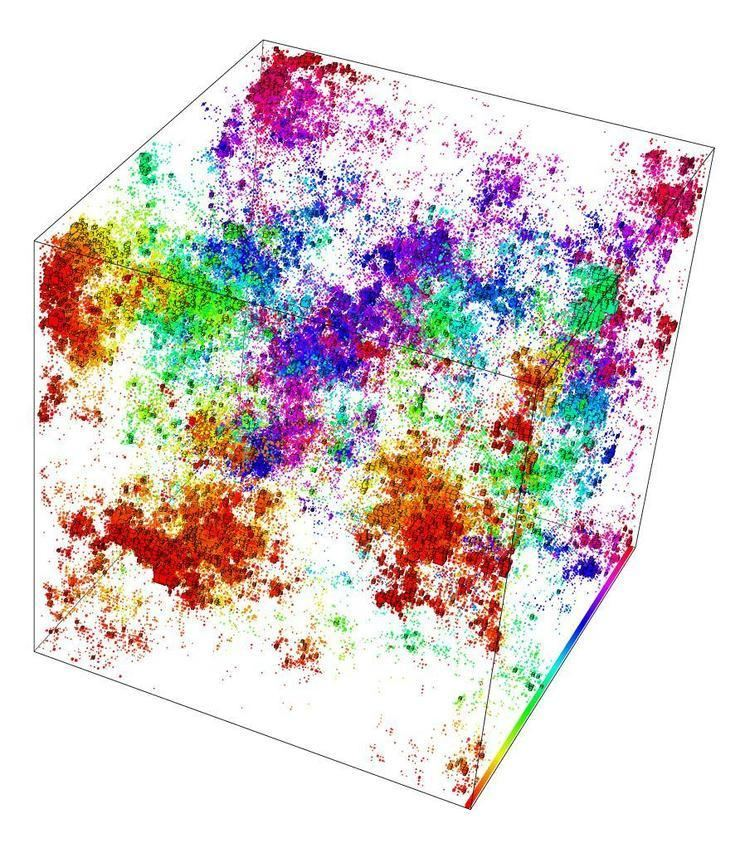Anderson localization

Updated onIn condensed matter physics, Anderson localization is the absence of diffusion of waves in a disordered medium. This phenomenon is named after the American physicist P. W. Anderson, who was the first one to suggest the possibility of electron localization inside a semiconductor, provided that the degree of randomness of the impurities or defects is sufficiently large.

Contents

Anderson localization is a general wave phenomenon that applies to the transport of electromagnetic waves, acoustic waves, quantum waves, spin waves, etc. This phenomenon is to be distinguished from weak localization, which is the precursor effect of Anderson localization (see below), and from Mott localization, named after Sir Nevill Mott, where the transition from metallic to insulating behaviour is not due to disorder, but to a strong mutual Coulomb repulsion of electrons.

Introduction

In the original Anderson tight-binding model, the evolution of the wave function ψ on the d-dimensional lattice Zd is given by the Schrödinger equation

i ψ ˙ = H ψ   ,

where the Hamiltonian H is given by

( H ϕ ) ( j ) = E j ϕ ( j ) + k j V ( | k j | ) ϕ ( k )   ,

with Ej random and independent, and interaction V(r) falling off as r−2 at infinity. For example, one may take Ej uniformly distributed in [−W,   +W], and

V ( | r | ) = { 1 , | r | = 1 0 , otherwise.

Starting with ψ0 localised at the origin, one is interested in how fast the probability distribution | ψ | 2 diffuses. Anderson's analysis shows the following:

• if d is 1 or 2 and W is arbitrary, or if d ≥ 3 and W/ħ is sufficiently large, then the probability distribution remains localized:
• uniformly in t. This phenomenon is called Anderson localization.
• if d ≥ 3 and W/ħ is small,
• n Z d | ψ ( t , n ) | 2 | n | D t   , where D is the diffusion constant.

Analysis

The phenomenon of Anderson localization, particularly that of weak localization, finds its origin in the wave interference between multiple-scattering paths. In the strong scattering limit, the severe interferences can completely halt the waves inside the disordered medium.

For non-interacting electrons, a highly successful approach was put forward in 1979 by Abrahams et al. This scaling hypothesis of localization suggests that a disorder-induced metal-insulator transition (MIT) exists for non-interacting electrons in three dimensions (3D) at zero magnetic field and in the absence of spin-orbit coupling. Much further work has subsequently supported these scaling arguments both analytically and numerically (Brandes et al., 2003; see Further Reading). In 1D and 2D, the same hypothesis shows that there are no extended states and thus no MIT. However, since 2 is the lower critical dimension of the localization problem, the 2D case is in a sense close to 3D: states are only marginally localized for weak disorder and a small spin-orbit coupling can lead to the existence of extended states and thus an MIT. Consequently, the localization lengths of a 2D system with potential-disorder can be quite large so that in numerical approaches one can always find a localization-delocalization transition when either decreasing system size for fixed disorder or increasing disorder for fixed system size.

Most numerical approaches to the localization problem use the standard tight-binding Anderson Hamiltonian with onsite-potential disorder. Characteristics of the electronic eigenstates are then investigated by studies of participation numbers obtained by exact diagonalization, multifractal properties, level statistics and many others. Especially fruitful is the transfer-matrix method (TMM) which allows a direct computation of the localization lengths and further validates the scaling hypothesis by a numerical proof of the existence of a one-parameter scaling function. Direct numerical solution of Maxwell equations to demonstrate Anderson localization of light has been implemented (Conti and Fratalocchi, 2008).

Experimental evidence

Two reports of Anderson localization of light in 3D random media exist up to date (Wiersma et al., 1997 and Storzer et al., 2006; see Further Reading), even though absorption complicates interpretation of experimental results (Scheffold et al., 1999). Anderson localization can also be observed in a perturbed periodic potential where the transverse localization of light is caused by random fluctuations on a photonic lattice. Experimental realizations of transverse localization were reported for a 2D lattice (Schwartz et al., 2007) and a 1D lattice (Lahini et al., 2006). Transverse Anderson localization of light has also been demonstrated in an optical fiber medium (Karbasi et al., 2012) and has also been used to transport images through the fiber (Karbasi et al., 2014). It has also been observed by localization of a Bose–Einstein condensate in a 1D disordered optical potential (Billy et al., 2008; Roati et al., 2008). Anderson localization of elastic waves in a 3D disordered medium has been reported (Hu et al., 2008). The observation of the MIT has been reported in a 3D model with atomic matter waves (Chabé et al., 2008). The MIT, associated with the nonpropagative electron waves has been reported in a cm sized crystal (Ying et al., 2016) Random lasers can operate using this phenomenon.

Comparison with diffusion

Standard diffusion has no localization property, being in disagreement with quantum predictions. However, it turns out that it is based on approximation of the principle of maximum entropy, which says that the probability distribution which best represents the current state of knowledge is the one with largest entropy. This approximation is repaired in Maximal Entropy Random Walk, also repairing the disagreement: it turns out to lead to exactly the quantum ground state stationary probability distribution with its strong localization properties.

Topics